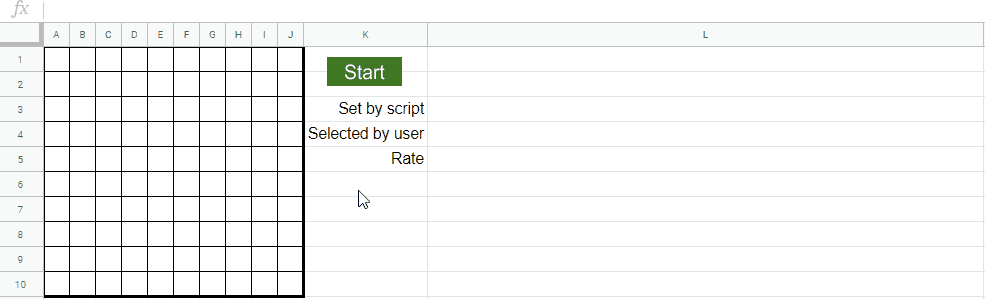# Characteristics of Response for onSelectionChange

Gists

## Abstract

I have already reported about “Change Tab Detection on Google Spreadsheet using onSelectionChange Event Trigger with Google Apps Script”. Ref It is considered that when the situation which uses the event trigger of onSelectionChange is thought, the response speed is important. So, here, I investigated the characteristics of response for the event trigger of onSelectionChange.

## Demo## Experiment

### Sample script

In order to investigate the response speed, I used the following sample script. The work of sample script can be seen at above demonstration movie. In this report, the script is important for discussing the result. So I pot this at this section instead of the appendix.

function start() {
const randRange = (a, b) => Math.floor(Math.random() * (b - a + 1) + a);
const getStr = () => "abcdefghij"[Math.floor(Math.random() * 10)];

const ca = CacheService.getScriptCache();
ca.remove("sample");
sheet.getRange("L3:L5").clearContent();
let ar = [];
for (let i = 0; i < 10; i++) {
const range = sheet.getRange(
getStr() + randRange(1, 10) + ":" + getStr() + randRange(1, 10)
);
ar.push(range.getA1Notation());
range.setBackground("#ff0000");

Utilities.sleep(3000); // <--- Important

range.setBackground("#ffffff");
}
const v = ca.get("sample").split(",");
const rr = ar.filter((r, i) => r == v[i]);
sheet
.getRange("L3:L5")
.setValues([
[JSON.stringify(ar)],
[JSON.stringify(v)],
[rr.length / ar.length],
]);
}

function onSelectionChange(e) {
const range = e.range;
const a1Notation = range.getA1Notation();
const ca = CacheService.getScriptCache();
const v = ca.get("sample");
ca.put(
"sample",
range.getBackgrounds().every((r) => r.every((c) => c == "#ff0000"))
? v
? v + "," + a1Notation
: a1Notation
: v
? v + ","
: ""
);
}


### Experimental procedure

In this script, the wait time of Utilities.sleep(3000) is very important for using the event trigger of onSelectionChange. 3000 means 3,000 ms. Because when range.setBackground("#ff0000") is run, it is required to retrieve the event object and run the script before range.setBackground("#ffffff") is run.

By the way, at GAS, the processing time is not stable as you know. So in this experiment, the average value for 20 times measurements was used for each data point which is shown by the following figure. At this time, the fluctuation of the average values was less than 5 %. I worry that each detailed-data point at my environment might be different from that at other user’s environment. But I think that the trend of this result can be used.

## Result and discussionFig. 1: Wait time vs. rate.

Figure 1 shows the wait tile vs. the rate. Rate means the successful rate that onSelectionChange could be correctly worked. From Fig. 1, it is found that when the wait time is small, the rate is 0 %. This indicates that the event object cannot be retrieved between range.setBackground("#ff0000") and range.setBackground("#ffffff"). When the wait time is 3,000 ms, the rate is closed to almost 100 %. By this, it is found that the wait time is very important for using the event trigger of onSelectionChange. And also, it is found that in order to retrieve the stable work of onSelectionChange, at least, the wait time of more than 3 seconds is required. Furthermore, from this experiment, it was found that the event trigger of onSelectionChange could be worked even when the other function was running.

## Summary

In this report, I investigated the characteristics of response for the event trigger of onSelectionChange. As the result, the following results were obtained.

1. It was found that the wait time was very important for using the event trigger of onSelectionChange.

2. It was found that in order to retrieve the stable work of onSelectionChange, at least, the wait time of more than 3 seconds was required.

3. It was found that the event trigger of onSelectionChange could be worked even when the other function was running.